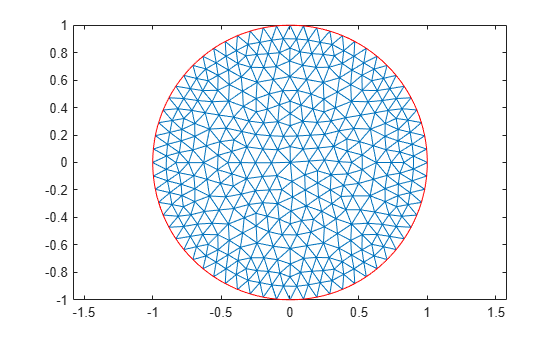Main Content

# Minimal Electric Potential

This example shows how to find the minimal electric potential by solving the equation

`$-\nabla \cdot \left(\epsilon \nabla \mathit{V}\right)=0$`

on the unit disk $\Omega =\left\{\left(\mathit{x},\mathit{y}\right)|{\mathit{x}}^{2}+{\mathit{y}}^{2}\le 1\right\}$, with $\mathit{V}\left(\mathit{x},\mathit{y}\right)={\mathit{x}}^{2}$ on the boundary $\partial \Omega$. Here, $\epsilon$ is the absolute dielectric permittivity of the material. The toolbox uses the relative permittivity of the material ${\epsilon }_{\mathit{r}}=\epsilon /{\epsilon }_{0}$, where ${\epsilon }_{0\text{\hspace{0.17em}}}$is the absolute permittivity of the vacuum. Note that the constant ${\epsilon }_{0\text{\hspace{0.17em}}}$does not affect the result in this example.

For the minimal surface problem, the value $\epsilon$ is given by

`$\epsilon =\frac{1}{\sqrt{1+{|\nabla \mathit{V}|}^{2}}}$`

Because the dielectric permittivity is a function of the solution V, the minimal surface problem is a nonlinear elliptic problem.

To solve the minimal surface problem, first create an electromagnetic model for electrostatic analysis.

`emagmodel = createpde('electromagnetic','electrostatic');`

Create the geometry and include it in the model. The `circleg` function represents this geometry.

`geometryFromEdges(emagmodel,@circleg);`

Plot the geometry with the edge labels.

```pdegplot(emagmodel,'EdgeLabels','on'); axis equal title 'Geometry with Edge Labels';```Specify the vacuum permittivity value in the SI system of units.

`emagmodel.VacuumPermittivity = 8.8541878128E-12;`

Specify the relative permittivity of the material.

```perm = @(region,state) 1./sqrt(1+state.ux.^2 + state.uy.^2); electromagneticProperties(emagmodel,'RelativePermittivity',perm); ```

Specify the electrostatic potential at the boundary using the function$\text{\hspace{0.17em}}\mathit{V}\left(\mathit{x},\mathit{y}\right)={\mathit{x}}^{2}$.

```bc = @(region,~)region.x.^2; electromagneticBC(emagmodel,'Voltage',bc,'Edge',1:emagmodel.Geometry.NumEdges); ```

Generate and plot the mesh.

```generateMesh(emagmodel); figure; pdemesh(emagmodel); axis equal```Solve the model.

```R = solve(emagmodel); V = R.ElectricPotential;```

Plot the electric potential, using the `Contour` parameter to display equipotential lines.

```figure; pdeplot(emagmodel,'XYData',V,'ZData',V,'Contour','on'); xlabel 'x' ylabel 'y' zlabel 'V(x,y)' title 'Minimal Electric Potential'```## SupportGet trial now